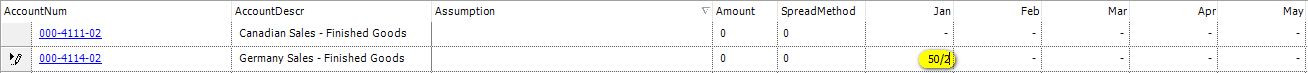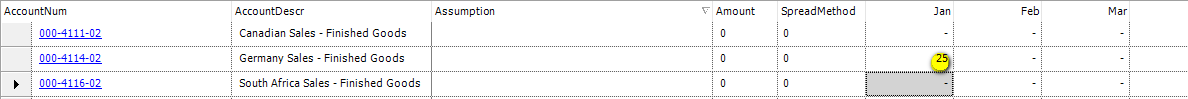Certain screens within the program has been setup with the ability to do basic math calculations within the cell.  The math functions are:

- Subtraction ("-");

- Multiplication ("*"); and

- Division ("/).

The below example uses the functionality embedded in the the Basic Budet Entry screen.As above, place your curser in the cell that you would like to do a calculation and enter the calculation.  In this example, we are dividing 50 by 2.

Then "Enter" to activate the calculation.  Please note below that the answer of 25 is now in the Jan cell.Please note that the formula (50/2) is not saved/retained - only the final answer (25) is saved/retained in the cell.